# CTheoreticalModel Class Reference

Theoretical Model derived in section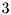, page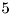. More...

#include <CTheoreticalModel.h>

List of all members.

## Public Member Functions

CTheoreticalModel (double alpha, double beta)
Construct a new model using the given parameters.
double algorithmA ()
calculates [some property] by implementing algorithmon pagevoid equationB (double gamma)
updates the model by [some parameter] according to the dynamics of equation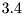on pagebool testC (double theta)
tests [some parameter] against the model; implements condition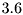## Private Attributes

double m_alpha
[parameter] defined in equation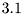double m_beta
[parameter] defined in equation## Detailed Description

Theoretical Model derived in section, page.

This is a detailed description of the model

## Constructor & Destructor Documentation

 CTheoreticalModel::CTheoreticalModel ( double alpha, double beta )

Construct a new model using the given parameters.

Parameters:
 [in] alpha [parameter] defined in equation[in] beta [parameter] defined in equation## Member Function Documentation

 double CTheoreticalModel::algorithmA ( )

calculates [some property] by implementing algorithmon pageReturns:
[some property]

 void CTheoreticalModel::equationB ( double gamma )

updates the model by [some parameter] according to the dynamics of equationon pageParameters:
 [in] gamma [parameter] defined in equation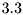bool CTheoreticalModel::testC ( double theta )

tests [some parameter] against the model; implements conditionParameters:
 [in] theta [some parameter] defined by equation## Member Data Documentation

 double CTheoreticalModel::m_alpha [private]

[parameter] defined in equationdouble CTheoreticalModel::m_beta [private]

[parameter] defined in equationThe documentation for this class was generated from the following file:

Generated on Sat Apr 17 20:56:58 2010 for dummy by1.5.8